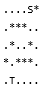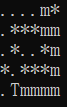• 深度优先搜素

Problem Description

There is a rectangular room, covered with square tiles. Each tile is colored either red or black. A man is standing on a black tile. From a tile, he can move to one of four adjacent tiles. But he can't move on red tiles, he can move only on black tiles.

Write a program to count the number of black tiles which he can reach by repeating the moves described above.

Input

The input consists of multiple data sets. A data set starts with a line containing two positive integers W and H; W and H are the numbers of tiles in the x- and y- directions, respectively. W and H are not more than 20.

There are H more lines in the data set, each of which includes W characters. Each character represents the color of a tile as follows.

'.' - a black tile
'#' - a red tile
'@' - a man on a black tile(appears exactly once in a data set)

Output

For each data set, your program should output a line which contains the number of tiles he can reach from the initial tile (including itself).

Sample Input

6 9
....#.
.....#
......
......
......
......
......
#@...#
.#..#.
11 9
.#.........
.#.#######.
.#.#.....#.
.#.#.###.#.
.#.#..@#.#.
.#.#####.#.
.#.......#.
.#########.
...........
11 6
..#..#..#..
..#..#..#..
..#..#..###
..#..#..#@.
..#..#..#..
..#..#..#..
7 7
..#.#..
..#.#..
###.###
...@...
###.###
..#.#..
..#.#..
0 0

Sample Output

45
59
6

13
在线翻译：
问题描述
有一个长方形的房间，满是方形的瓷砖。每一瓦都是红色或黑色的。一个人正站在一个黑色的瓷砖上。从一个瓷砖，他可以移动到四个相邻的瓷砖之一。但他不能在红色的瓷砖，他只能移动黑色瓷砖。
写一个程序来计算他可以通过重复上面描述的移动的黑色瓷砖的数量。
输入
输入由多个数据集组成。数据集从一行包含两个正整数的行开始，而在两个方向上，分别是瓷砖的数量和。没有超过20个。
数据集上有更多的行，其中每一个都包含了字符。每个字符表示一个瓷砖的颜色如下。
“-一个黑色的瓦
“# -红瓦
在一个黑色的瓷砖上，一个男人在一个数据集里出现了一次
输出
对于每一个数据集，您的程序应该输出一行，其中包含的数量，他可以从最初的瓷砖（包括本身）。

解题思路：搜索（深度优先搜素法）
1.循环+条件判断；
2.函数的调用+递归；
AC代码：

#include <stdio.h>
#include <string.h>
int n,m,cnt,x,y;
char s;
int to = {{1,0},{0,1},{-1,0},{0,-1}};       //根据题意定义二维数组， 查询四周位置；
void dfs(int i,int j)
{
cnt++;                                       //记录符合条件的个数；
s[i][j] = '#';                               //首先确定型号，便于下文的查询；
for(int k = 0; k<4; k++)
{
x = i+to[k];
y = j+to[k];                          //四周位置定位；
if(x<n && y<m && x>=0 && y>=0 && s[x][y] == '.')
dfs(x,y);                             //函数递归，直到查询到结果停止；
}
return;                                       //void无输出；
}
int main()
{
int i,j,fi,fj;
while(~scanf("%d%d%*c",&m,&n))               //%*c输出空格，消除影响；
{
if(m == 0 && n == 0)                     //特殊情况;
break;
for(i = 0; i<n; i++)
{
for(j = 0; j<m; j++)
{
scanf("%c",&s[i][j]);
if(s[i][j] == '@')              //开始条件；
{
fi = i;
fj = j;
}
}
getchar();                          //吸收还行空格；
}
cnt = 0;                                //新的样例，重新归零；
dfs(fi,fj);                             //直接调用函数；
printf("%d\n",cnt);
}
    return 0;
}
</pre><pre name="code" class="html"><pre name="code" class="html">
#include<cstdio>
#include<cstdlib>
#include<iostream>                   //c++数据的输出和输入；
#include<cstring>
using namespace std;
int m,n,cnt;
char s;
int dfs(int i, int j)
{
//cnt++;
if(i<1 || i>n || j<1 || j>m)
return 0;
if(s[i][j]!='#')
{
s[i][j]='#';
return 1+dfs(i-1,j)+dfs(i+1,j)+dfs(i,j-1)+dfs(i,j+1);     //函数递归，累加返回值；
}
else
return 0;
}
int main ()
//(int agrc,char *argv[])
{
while(cin>>m>>n)
{
if(m==0&&n==0)
break;
int i,j;
for(i=1; i<=n; i++)
for(j=1; j<=m; j++)
cin>>s[i][j];
for(i=1; i<=n; i++)
for(j=1; j<=m; j++)
if(s[i][j]=='@')          //确定查询的起始位置；
cout<<dfs(i,j)<<endl;
}
return 0;
//return EXIT_SUCCESS;
}
总结： 明白题意；注意细节；


展开全文• 深度优先搜素（DFS）：如果当前节点有孩子，遍历当前孩子再遍历依次兄弟节点的孩子。 <div class="root"> <div class="items"> <ol> <li class="list-item">a</li> </ol&...
深度优先搜素（DFS）：如果当前节点有孩子，遍历当前孩子的孩子再遍历兄弟节点的孩子（一条路走到头再回来走另一条）。
**HTML 代码**
<div class="root">
<div class="items">
<ol>
<li class="list-item">a</li>
</ol>
<div class="item">
<span>aaa</span>
</div>
</div>
</div>

// 深度优先搜索 递归版
<script>
function DFS(node, list) {
if (node) {
list.push(node);
var child = node.children;
// 如果存在孩子，对每个孩子再进行递归搜索
for (var i = 0; i < child.length; i++) {
DFS(child[i], list);
}
}
return list;
}
var root = document.querySelector('.root');
var list = [];
console.log(DFS(root, list));
</script>

输出为： [div.root, div.items, ol, li.list-item, div.item, span]
广度优先搜索：先遍历根节点的所有孩子，再遍历所有孩子的孩子（一层一层的往外走）
function BFS(node) {
// 分别定义两个数组，result 用来存放最后结果；arr 模拟先进先出队列
var result = [];
var arr = [];
if (node) {
arr.push(node);
while(arr.length) {
// 每次取出队列中的第一个元素，并将该元素的孩子 push 进队列
var item = arr.shift();
var children = item.children;
result.push(item);
for (var i = 0; i < children.length; i++) {
arr.push(children[i]);
}
}
}
return result;
}
var node = document.querySelector('.root');
console.log(BFS(node));

输出为： [div.root, div.items, ol, div.item, li.list-item, span]
扩展： 输入一颗二叉树的跟节点和一个整数，打印出二叉树中结点值的和为输入整数的所有路径。路径定义为从树的根结点开始往下一直到叶结点所经过的结点形成一条路径。(注意: 在返回值的list中，数组长度大的数组靠前) 用例: {10,5,12,4,7},22
对应输出应该为:
[[10,5,7],[10,12]]
/* function TreeNode(x) {
this.val = x;
this.left = null;
this.right = null;
} */
function FindPath(root, expectNumber)
{
// write code here
// 深度优先搜索求和问题
var result = [];
if (!root) {
return result;
}
DFS(root, expectNumber, [], 0, result);
return result;
}

function DFS(root, expectNumber, path, currentSum, result) {
currentSum += root.val;
path.push(root.val);
if (currentSum === expectNumber && root.left === null && root.right === null) {
result.push(path.slice(0));
}
if (root.left) {
DFS(root.left, expectNumber, path, currentSum, result);
}
if (root.right) {
DFS(root.right, expectNumber, path, currentSum, result);
}
// 每次清空数组
path.pop();
}

展开全文广度优先搜索
• 使用简单的深度优先搜素来寻找迷宫的出口， 并显示正确的路径。 现有一个由各种符号组成的简单迷宫地图 ‘S' ：起点 ’T ‘：终点 ‘ * ’：障碍 #include <iostream> #include <string> using ...
使用简单的深度优先搜素来寻找迷宫的出口， 并显示正确的路径。
现有一个由各种符号组成的简单迷宫地图‘S' ：起点    ’T ‘：终点    ‘ * ’：障碍
#include <iostream>
#include <string>
using namespace std;
int n, m;                  //n为行， m为列
string maze;
bool vis;
int dir = { {-1,0}, {0,-1}, {1,0}, {0,1}};    //可移动的四个方向
bool in(int x, int y) {
return 0 <= x && x < n && 0 <= y && y < m;       //判断是否在迷宫内
}
bool dfs(int x, int y) {
if(maze[x][y] == 'T'){              //若到终点， 则返回
return true;
}
vis[x][y] = 1;                    //每走一步就标记
maze[x][y] = 'm';

for(int i=0; i<4; i++){            //上下左右，都走一遍
int tx = x + dir[i];
int ty = y + dir[i];
if(in(tx, ty) && maze[tx][ty] != '*' && !vis[tx][ty]){
if(dfs(tx, ty)){
return true;     //若下一步在迷宫内，不是障碍物， 也不是走过的路，就递归
}
}
}

//回溯
vis[x][y] = 0;
maze[x][y] = '.';
return false;
}
int main() {
cin >> n >> m;
for (int i = 0; i < n; i++) {
cin >> maze[i];
}
int x, y;
for (int i = 0; i < n; i++) {         //两个for循环找到终点‘T’的坐标
for (int j = 0; j < m; j++) {
if (maze[i][j] == 'S') {
x = i, y = j;
}
}
}
if (dfs(x, y)) {                    //如果找到就输出路径， 没找到打印NO
for (int i = 0; i < n; i++) {
cout << maze[i] << endl;
}
} else {
cout << "NO!" << endl;
}
return 0;
}
输入n, m后， 结果会让你满意， 使用符号m打印路径。展开全文• 搜索 （深度优先搜素
 Oil Deposits
Time Limit: 2000/1000 MS (Java/Others) Memory Limit: 65536/32768 K (Java/Others) Total Submission(s): 8274 Accepted Submission(s): 4860

Problem Description The GeoSurvComp geologic survey company is responsible for detecting underground oil deposits. GeoSurvComp works with one large rectangular region of land at a time, and creates a grid that divides the land into numerous square plots. It then analyzes each plot separately, using sensing equipment to determine whether or not the plot contains oil. A plot containing oil is called a pocket. If two pockets are adjacent, then they are part of the same oil deposit. Oil deposits can be quite large and may contain numerous pockets. Your job is to determine how many different oil deposits are contained in a grid.

Input The input file contains one or more grids. Each grid begins with a line containing m and n, the number of rows and columns in the grid, separated by a single space. If m = 0 it signals the end of the input; otherwise 1 <= m <= 100 and 1 <= n <= 100. Following this are m lines of n characters each (not counting the end-of-line characters). Each character corresponds to one plot, and is either *', representing the absence of oil, or @', representing an oil pocket.

Output For each grid, output the number of distinct oil deposits. Two different pockets are part of the same oil deposit if they are adjacent horizontally, vertically, or diagonally. An oil deposit will not contain more than 100 pockets.

Sample Input 1 1 * 3 5 *@*@* **@** *@*@* 1 8 @@****@* 5 5 ****@ *@@*@ *@**@ @@@*@ @@**@ 0 0
Sample Output 0 1 2
2
在线翻译：
石油矿床 时间限制：2000 / 1000毫秒（java /其他）内存限制：65536 / 32768 K（java /其他） 提交总提交：8274接受提交：4860 问题描述 的geosurvcomp地质调查公司负责探测地下石油储量。geosurvcomp运作在一个大的矩形区域的土地在一个时间，并创建一个网格把土地分成很多广场地块。然后分别对每一个小区分别进行分析，利用传感设备确定小区是否含有油。被称为口袋的阴谋。如果两个口袋是相邻的，那么他们是相同的石油存款的一部分。石油储量相当大，而且可能蕴藏大量的石油储量。你的工作是确定在一个网格中包含多少不同的石油储量。 输入 输入文件包含一个或多个网格。每一个网格开始一行包含米和氮，在网格中的行和列的数目，用一个空格隔开。如果米= 0，它的信号的输入端，否则1个< = = 100和1。下面这是每个字符的行（不算行字符的结束）。每个字符对应一个图，并且是' * '，代表没有油，或' @ '，代表一个石油口袋。 输出 对于每一个网格，输出的数量不同的石油储量。如果他们是相邻的水平，垂直或对角的相同的油存款的一部分，2个不同的口袋是相同的。一个石油储量将不包含超过100个口袋。
解题思路；
1.深度优先搜素
2.queue的使用
#include<stdio.h>
#include<queue>
using namespace std;
char map;       //存图；
int n,m;
int dir={-1,-1,0,-1,1,-1,-1,0,1,0,-1,1,0,1,1,1}; //八个方向,无小括号；
struct state
{
int x,y;
};
void bfs(int i,int j)
{
int k;
map[i][j]='*';
queue<state>q;         //建立一个以state这个数据类型为节点的队列，队列的变量为q;
state cur,next;        //数据结构中下个节点；
cur.x=i;
cur.y=j;
q.push(cur);           //queue中 进队列；
while(!q.empty())      //循环的条件 不为空；
{
cur=q.front();     //得到队首的值
q.pop();           //queue中 出队列；
for(k=0;k<8;k++)
{
next.x=cur.x+dir[k];
next.y=cur.y+dir[k];
if(next.x>=0&&next.x<m&&next.y>=0&&next.y<n)
{
if(map[next.x][next.y]=='@')
{
map[next.x][next.y]='*';
q.push(next);       //queue中 进队列再次执行，直到最后；
}
}
}
}
}
int main()
{
int i,j,sum=0;
while(scanf("%d%d",&m,&n),m)
{
getchar();             //消除字符空格；
sum=0;                 //循环中变量的初始化；
for(i=0;i<m;i++)
gets(map[i]);
for(i=0;i<m;i++)
for(j=0;j<n;j++)
if(map[i][j]=='@')
{
sum++;
bfs(i,j);          //调用函数；
}
printf("%d\n",sum);
}
return 0;
}
<pre name="code" class="html">#include <iostream>
#include <queue>
using namespace std; //这几个头文件必不可少

int main()
{
queue<类型（如int）> q; //使用前需定义一个queue变量，且定义时已经初始化
while(!q.empty()) q.pop(); //重复使用时，用这个初始化
q.push(1); //进队列
q.pop(); //出队列
int v=q.front(); //得到队首的值
int s=q.size(); //得到队列里元素个数


展开全文• 深度优先搜素算法题与分析 题目如下 小朋友 A 在和 ta 的小伙伴们玩传信息游戏，游戏规则如下： 有 n 名玩家，所有玩家编号分别为 0 ～ n-1，其中小朋友 A 的编号为 0 每个玩家都有固定的若干个可传信息的其他玩家...算法 java 动态规划
• 深度优先搜索属于图算法的一种,英文缩写为DFS即Depth First Search.其过程简要来说是对每一个可能的分支路径深入到不能再深入为止,而且每个节点只能访问一次.
• 深度优先搜素的关键在于（当下）一步怎么走，而下一步与（当下）一步怎么走是一样的。所以我们经常利用递归操作来进行。 全排列作为深度优先中的一道基础题，代码如下。（C语言实现） #include<stdio.h> #...c++
• 描述了深度优先搜索的定义，用法，及其一些经典的题型，带有图片，更加的生动形象。具体有数字的全排列这个问题，以及经典的走迷宫问题及其代码。
• 深度优先搜索 1. 搜索是指已知初始状态和目标状态，对问题的中间状态进行枚举的遍历的一种算法，通俗的讲，搜索就是比较复杂的枚举。 2.深度优先搜索是按照深度的方式进行搜索，就是把所有可行的方案都列举出来，...
• 深度优先搜素，找具体两个顶点的最短路径 void min_path_dfs(Graph *pg,int cur,int end,int curpath,int mark[],int *pminpath,int path[],int cnt,int savepath[],int *savecnt){ if(curpath>*pminpath){//还...
• 64-linux-gnu/9/../../../../include/c++/9/bits/stl_vector.h:1043:34 往往是由于下标出现了负数，在使用深度优先搜索时，书写约束条件不小心把vectorp[i][j]!=目标，写在第一个条件时，可能在使用dfs递归时出现...
• 题目描述 如图，A 点有一个过河卒，需要走到目标 B 点。卒行走规则：可以向下、或者向右。同时在棋盘上的任一点有一个对方的马（如上图的C点），该马所在的点和所有跳跃一步可达的点称为对方马的控制点。...CodeVS
• class Solution { vectorp; int ans=0; void dfs(vector>& land,int i,int j) { if(i||j||i>=land.... 在定义深度优先搜索时，当不是0时要退出。 进行八个方向的搜索。 在计算一次水域大小后，要对水域大小重新赋值为0.
• 搜索算法分为广度优先搜索（BFS）和深度优先搜索（DFS）。 广度优先搜索 基本思想：从初始状态S 开始，利用规则，生成所有可能的状态。一层层的寻找所要的结果，如果没找到所需要的答案，就继续将这层寻找完，...
• 配合思考题 bfs 广度优先搜索 小易总是感觉饥饿，所以作为章鱼的小易经常出去寻找贝壳吃。最开始小易在一个初始位置x_0。对于小易所处的当前位置x，他只能通过神秘的力量移动到 4 * x + 3或者8 * x + 7。因为使用...
• 圆环由n个圆组成，如图所示。将自然数1、2，…，n分别放入每个圆，两个相邻圆中的数字总和应为质数。 注意：第一个圆的数目应始终为1。 n（0 <n <20）。 输出格式如下所示。每一行代表环中从顺时针和逆时针1...
• c语言dfs基础入门题目 n皇后问题 n-皇后问题是指将 n 个皇后放在 n∗n 的国际象棋棋盘上，使得皇后不能相互攻击到，即任意两个皇后都不能处于同一行、同一列或同一斜线上。 现在给定整数n，请你输出所有的满足条件的...dfs 算法 c语言
• #include <bits/stdc++.h> #define VSIZE 100 int visitde[VSIZE]; typedef int VType; //顶点数据类型 typedef int EType; //边的数据类型 typedef struct AdjMGrahp{ VType vexs[VSIZE];...
• 二维数组地图的寻路最短步数 （下标从1开始） 0 0 1 0 0 0 0 0 0 0 1 0 0 1 0 0 ...这样的一个5*4的矩阵，1代表路障，0可以走，从（1，1）出发，到（4，3）的位置 ...也可以用广度优先搜索做
• The GeoSurvComp geologic survey company is responsible for detecting underground oil deposits. GeoSurvComp works with one large rectangular region of land at a time, and creates a grid that divides th...
• void dfs(int step){ //深度优先搜索 int i; if(step>x) //当x个位置已经确定后，开始判断是否符合条件 jude(); for(i=1;i;i++){ if(book[i]==0){ //如果此位置没有标记 book[i]=1; //标记...
• 使用一个长度为N的数组，1 本题目已给出main函数，需要以递归方式在头文件DFS.h中实现DFS函数，函数没有返回值，整个搜索过程的输出均在DFS函数中实现，函数有4个参数...输出：深度搜索的过程，每个数字以空格间隔，
• 题目描述  已知 n 个整数 x1,x2,…,xn，以及一个整数 k（k）。从 n 个整数中任选 k 个整数相加，可分别得到一系列的和。例如当 n=4，k＝3，4 个整数分别为 3，7，12，19 时，可得全部的组合与它们的和...dfs NOIP
• #include &lt;iostream&gt; #include &lt;algorithm&gt; #include &lt;cstdio&gt; using namespace std; int mindis = 9999999 ; const int MAX = 1000 ; ...void init...
• 感觉想学会DFS说到底最重要的也就是弄懂递归怎么用 #include<stdio.h> #define X 8 #define Y 8 int chess[X][Y] = { 0 };//棋盘 bool nextxy(int* x, int* y, int count) {//x，y使用指针类型是为了直接修改...算法 C语言
• 实例化图，并使用深度优先搜索、广度优先搜索 创建树，新建边 var graph = new Graph( 10 ) ; graph .addEdge ( 0 , 1 ) ; graph .addEdge ( 0 , 2 ) ; graph .addEdge ( 0 , 5 ) ; graph ....javascript 算法...